Question

On a cold winter day, the outside temperature is -20°C and the inside temperature is maintained at 20°C. There is a net heat flow to the outside through the walls, roof, etc., of 25 kW. What is the rate of increase of the entropy of the universe as a result of this heat flow?

Okay,

so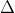Shot = 25000 / 293 = 85.3 W/KScold = 25000 / 253 = 98.8 W/K

Therefore,

rate of increase of the entropy of the universeS = 98.8 - 85.3S = 13 W/K

#### Earn Coins

Coins can be redeemed for fabulous gifts.

Similar Homework Help Questions
• ### On a cold day, 26100 J of heat leaks out of a house. The inside temperature is 22 °C, and the outside temperature is -20...

On a cold day, 26100 J of heat leaks out of a house. The inside temperature is 22 °C, and the outside temperature is -20 °C. What is the increase in the entropy of the universe that this heat loss produces?

• ### On a cold winter day, the outside temperature is –5.0 °C while the interior of a...

On a cold winter day, the outside temperature is –5.0 °C while the interior of a well-insulated garage is maintained at 20.0 °C by an electric heater. Assume the walls have a total area of 75 m2, a thickness of 0.15 m, and a thermal conductivity of 0.042 W/(m · C°). What is the cost to heat the garage for six hours at these temperatures if the cost of electricity is \$ 0.11/kWh? Note: 1 kWh = 3.6 × 106...

• ### On a cold winter day where the outside temperature is -10o C, a heat pump provides...

On a cold winter day where the outside temperature is -10o C, a heat pump provides 20 kW to heat a house to 20o C. The heat pump has a COPHP of 4 at the maximum power. The next day a storm brings the outside temperature down to −15o C. If the ratio of COPHP and the reversible COPrev for day 1 and day 2 is the same, COPHP for both days is the same, and that the house loses...

• ### The temperature difference between the inside and the outside of a building on a cold winter...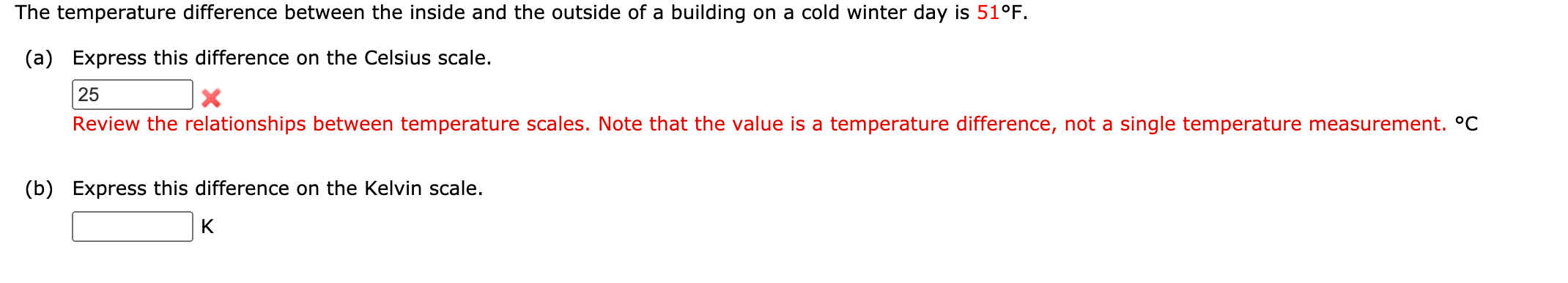The temperature difference between the inside and the outside of a building on a cold winter day is 51°F. (a) Express this difference on the Celsius scale. 25 x Review the relationships between temperature scales. Note that the value is a temperature difference, not a single temperature measurement. °C (b) Express this difference on the Kelvin scale. K

• ### A heat pump is to be used to heat a house in winter and then reversed...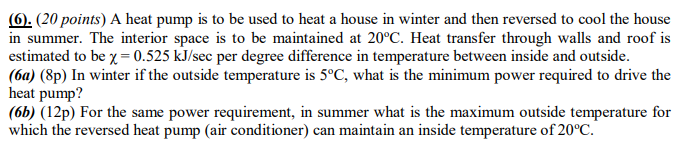A heat pump is to be used to heat a house in winter and then reversed to cool the house in summer. The interior space is to be maintained at 20oC. Heat transfer through walls and roof is estimated to be χ = 0.525 kJ/sec per degree difference in temperature between inside and outside. (6a) (8p) In winter if the outside temperature is 5oC, what is the minimum power required to drive the heat pump? (6b) (12p) For the same...

• ### 5.75 A heating system must maintain the interior of a building at TH = 20 degree C when the outside temperature is T =...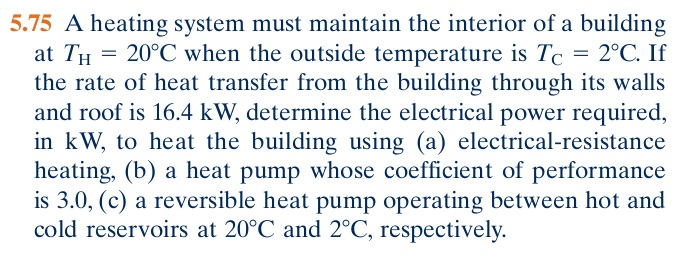5.75 A heating system must maintain the interior of a building at TH = 20 degree C when the outside temperature is T = 2 degree C. If the rate of heat transfer from the building through its walls and roof is 16.4 kW, determine the electrical power required, in kW, to heat the building using (a) electrical-resistance heating, (b) a heat pump whose coefficient of performance is 3.0, (c) a reversible heat pump operating between hot and cold reservoirs...

• ### On a winter day with a temperature of Tc, the temperature inside a room is Th,...

On a winter day with a temperature of Tc, the temperature inside a room is Th, where Th > Tc. The heat conduction rate through a slab of thickness L, surface area A, thermal conductivity k is measured to be H. Assume you found a way to triple the slab thickness and reduce the thermal conductivity to one quarter, what is the new heat transfer rate H’ in terms of H? Assume the temperatures inside and outside the room and...

• ### help please Chapter 15, Problem 078 GO On a cold day, 28000 J of heat leaks...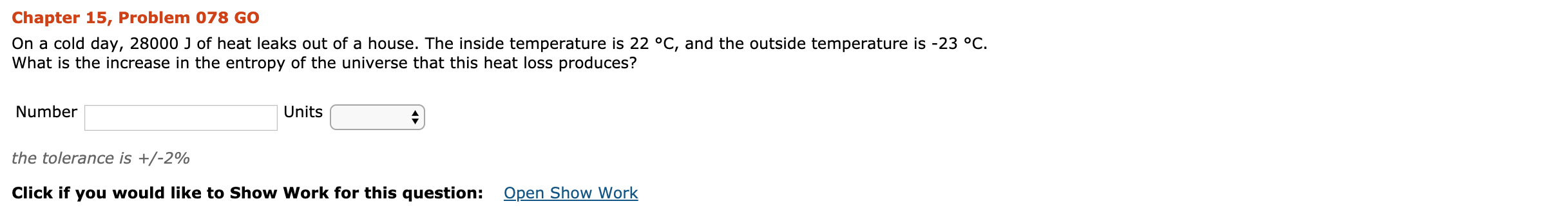help please Chapter 15, Problem 078 GO On a cold day, 28000 J of heat leaks out of a house. The inside temperature is 22 °C, and the outside temperature is -23 °C. What is the increase in the entropy of the universe that this heat loss produces? Number Units the tolerance is +/-2% Click if you would like to Show Work for this question: Open Show Work

• ### In this problem you will estimate the heat lost by a typical house, assuming that the temperature inside is T(in) = 20 degrees celcius and the temperature outside is T(out) = 0 degrees celcius

In this problem you will estimate the heat lost by a typical house, assuming that the temperature inside is T(in) = 20 degrees celcius and the temperature outside is T(out) = 0 degrees celcius. The walls have fiberglass insulation, which dominates the heat conduction properties of the wall So we can consider the wall to have a thermal conductivity of k(wall) = 0.048 W/m/K . We will take the thickness of the walls and ceiling to be L(wall) = 12...

• ### ature di e between the inside and the outside of a b on a cold winter...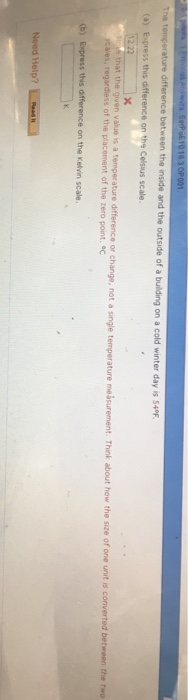ature di e between the inside and the outside of a b on a cold winter day is 549F (a) Express this difference on the Celsius scale that the given value is a temperature difference or change, not a single temperature messurement. hink about how the size of one unit is converted be the two of the placement of the zero point. °c on the Kelvin scale Need Help?